Identity Z in the sequence of reactions,

CH3CH2CH=CH2 $\stackrel{\mathrm{HBr}/{\mathrm{H}}_{2}{\mathrm{O}}_{2}}{\to }$Y$\stackrel{{\mathrm{C}}_{2}{\mathrm{H}}_{5}\mathrm{ONa}}{\to }$Z

(a) CH3-(CH2)3-O-CH2CH3

(b) (CH3)2CH2-O-CH2CH3

(c) CH3(CH2)4-O-CH3

(d) CH3CH2-CH(CH3)-O-CH2CH3

Concept Questions :-

Ethers: Preparation, Physical & Chemical Properties, Uses
High Yielding Test Series + Question Bank - NEET 2020

Difficulty Level:

Reaction of ethyl formate with excess of CH3MgI followed by hydrolysis gives

1. n-propyl alcohol

2. ethanol

3. isopropyl alcohol

4.  propanal

Concept Questions :-

Alcohols: Preparation, Physical and Chemical Properties
High Yielding Test Series + Question Bank - NEET 2020

Difficulty Level:

In a reaction,

${\mathrm{CH}}_{2}={\mathrm{CH}}_{2}\underset{\mathrm{acid}}{\overset{\mathrm{Hypochlorous}}{\to }}$M$\stackrel{\mathrm{R}}{\to }$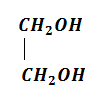M=molecules, R=reagent. M and R are

(a) ${\mathrm{CH}}_{3}{\mathrm{CH}}_{2}\mathrm{Cl}$ and NaOH

(b)  and aq. ${\mathrm{NaHCO}}_{3}$

(c) ${\mathrm{CH}}_{3}{\mathrm{CH}}_{2}\mathrm{OH}$ and HCl

(d)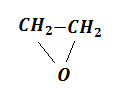and heat

Concept Questions :-

Alcohols: Preparation, Physical and Chemical Properties
High Yielding Test Series + Question Bank - NEET 2020

Difficulty Level:

Lucas reagent is

1. conc. HCl and anhy. ZnCl2

2. conc. HNO3 and anhy. ZnCl2

3. conc. HCl and hydrous ZnCl2

4. conc. HNO3 and hydrous ZnCl2

Concept Questions :-

Alcohols: Preparation, Physical and Chemical Properties
High Yielding Test Series + Question Bank - NEET 2020

Difficulty Level:

The compound which reacts fastest with Lucas reagent is (at room temperature)

(a) butan-1-ol

(b) butan-2-ol

(c) 2-methyl propan-1-ol

(d) 2-methyl propan-2-ol

Concept Questions :-

Alcohols: Preparation, Physical and Chemical Properties
High Yielding Test Series + Question Bank - NEET 2020

Difficulty Level:

The ionisation constant of phenol is higher than that of ethanol because

1. phenoxide ion is bulkier than ethoxide

2. phenoxide ion is stronger base than ethoxide

3. phenoxide ion is stabilised through delocalisation

4. phenoxide ion is less stable than ethoxide

Concept Questions :-

Phenols: Preparation, Properties, Acidic Nature of Phenol
High Yielding Test Series + Question Bank - NEET 2020

Difficulty Level:

The general molecular formula, which represents the homologous series of alkanols is

(a) CnH2nO2        (b) CnH2nO

(c) CnH2n+1O       (d) CnH2n+2O

Concept Questions :-

Alcohols: Preparation, Physical and Chemical Properties
High Yielding Test Series + Question Bank - NEET 2020

Difficulty Level:

$\mathrm{R}-\mathrm{COOH}\to {\mathrm{RCH}}_{2}\mathrm{OH}$. This reduction cannot be done  by:

(a) ${\mathrm{NaBH}}_{4}$                               (b) Na + alcohol

(c) ${\mathrm{LiAlH}}_{4}$                                (d) all of these

Concept Questions :-

Alcohols: Preparation, Physical and Chemical Properties

Difficulty Level:

What mass of isobutylene is obtained from 37 g of tertiary butyl alcohol by heating with 20% H2SO4 at 363 K, if the yield is 65%?

1. 16 g

2. 18.2 g

3. 20 g

4. 22 g

Concept Questions :-

Alcohols: Preparation, Physical and Chemical Properties
High Yielding Test Series + Question Bank - NEET 2020

Difficulty Level:

Glycol is prepared industrially by the following reactions:

(a)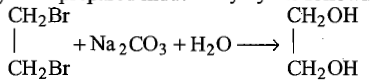(b)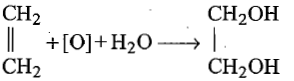(c)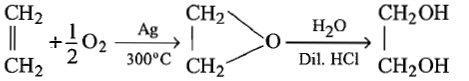(d) none of the above

Concept Questions :-

Alcohols: Preparation, Physical and Chemical Properties